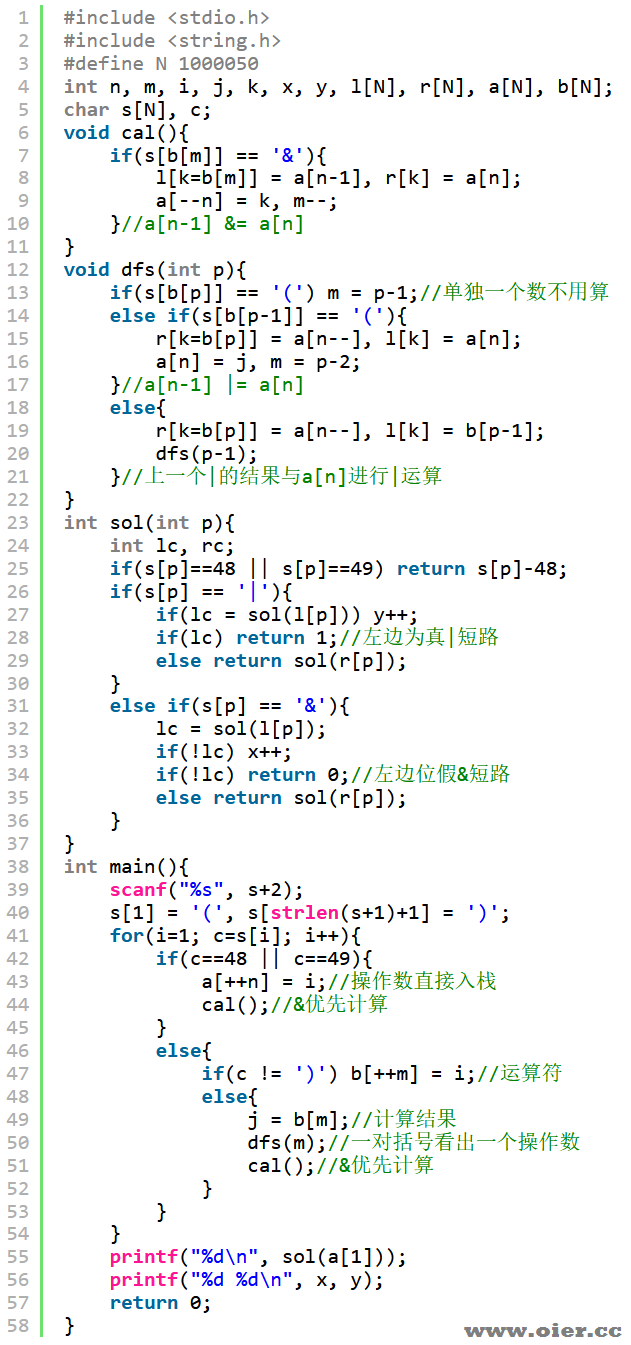839+

## 题目描述

$0 \mathbin{\&} 0 = 0 \mathbin{\&} 1 = 1 \mathbin{\&} 0 = 0$，$1 \mathbin{\&} 1 = 1$；
$0 \mathbin{|} 0 = 0$，$0 \mathbin{|} 1 = 1 \mathbin{|} 0 = 1 \mathbin{|} 1 = 1$。

## 输入输出样例

### 输入样例 #1

0&(1|0)|(1|1|1&0)


### 输出样例 #1

1
1 2


### 输入样例 #2

(0|1&0|1|1|(1|1))&(0&1&(1|0)|0|1|0)&0


### 输出样例 #2

0
2 3


## 说明

**【样例解释 \#1】**

“plain
0&(1|0)|(1|1|1&0)
=(0&(1|0))|((1|1)|(1&0)) //用括号标明计算顺序
=0|((1|1)|(1&0)) //先计算最左侧的 &，是一次形如 a&b 的“短路”
=0|(1|(1&0)) //再计算中间的 |，是一次形如 a|b 的“短路”
=0|1 //再计算中间的 |，是一次形如 a|b 的“短路”
=1
“

**【样例 \#3】**

**【样例 \#4】**

**【数据范围】**

| 测试点编号 | $\lvert s \rvert \le$ | 特殊条件 |
|:-:|:-:|:-:|
| $1 \sim 2$ | $3$ | 无 |
| $3 \sim 4$ | $5$ | 无 |
| $5$ | $2000$ | 1 |
| $6$ | $2000$ | 2 |
| $7$ | $2000$ | 3 |
| $8 \sim 10$ | $2000$ | 无 |
| $11 \sim 12$ | ${10}^6$ | 1 |
| $13 \sim 14$ | ${10}^6$ | 2 |
| $15 \sim 17$ | ${10}^6$ | 3 |
| $18 \sim 20$ | ${10}^6$ | 无 |

**【提示】**

– 字符串 0 和 1 是符合规范的；
– 如果字符串 s 是符合规范的，且 s 不是形如 (t) 的字符串（其中 t 是符合规范的），那么字符串 (s) 也是符合规范的；
– 如果字符串 a 和 b 均是符合规范的，那么字符串 a&b、a|b 均是符合规范的；
– 所有符合规范的逻辑表达式均可由以上方法生成。

## 程序实现